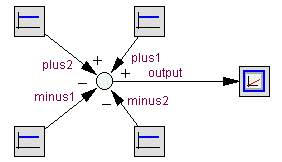﻿ 20-sim webhelp > Library > Signal > Block Diagram > plusminus

# plusminus

## Library

Signal\Block Diagram , System

## Use

Domains: Continuous, Discrete. Size: [n,m]. Kind: Block Diagrams.

## Description

This model yields a subtraction and/or summation of one or more input signals. While connecting input signals, 20-sim will ask whether the signals should be added or subtracted. The signals may have either size, but the size of all connected signals should be equal.For example the PlusMinus model can have 2 signals connected that are added and 2 signals that must be subtracted. 20-sim treats them as an array plus and an array minus with:

plus = [plus1;plus2];

and

minus = [minus1;minus2];

The output will then be equal to:

output = plus1 + plus2 - minus1 - minus2;

## Interface

 Inputs Description plus[any] minus[any] Any number of input signals (each of the same size) may be connected to be added (plus) or subtracted (minus). Outputs output

## Limitations

The signals connected to this model should all have the same size.